# AP Chemistry : Thermochemistry and Kinetics

## Example Questions

### Example Question #8 : Calorimetry, Specific Heat, And Calculations

You want to prepare a cup of tea. To do so, you pour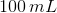of tap water at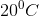in a cup that does not absorb microwave radiation and heat it in a microwave oven at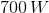of power. If you assume a density of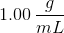for the water and know that its specific heat capacity is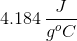, what time do you need to set in the microwave oven to heat the water to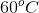?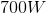of power is not enough to heat that a amount of water to the desired temperature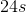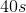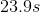Explanation: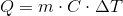Since the density of water is, the mass ofis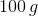. Plug in known values to the equation and solve.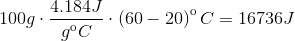Use the formula below to find the time needed to heat up the sample of water in the microwave: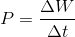Our answer must contain three significant figures.

### Example Question #9 : Calorimetry, Specific Heat, And Calculations

How much heat is needed to raisegrams of aluminum by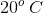?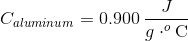.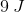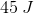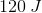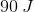Explanation:

To find the amount of heat needed to change the temperature of a given material by a certain amount, we'll need to use the equation for specific heat. The specific heat capacity of a compound represents the amount of energy necessary to raisegram of that substance by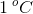.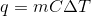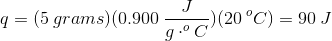### Example Question #10 : Calorimetry, Specific Heat, And Calculations

A 20g sample of iron at a temperature of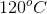is placed into a container of water. There are 300 milliliters of water in the container at a temperature of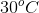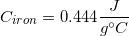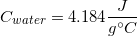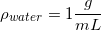What is the final temperature of the water?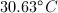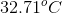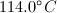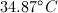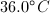Explanation:

There are two things to note before solving for the final temperature.

1. The density of water allows us to say that 300 milliliters of water is the same thing as 300 grams of water.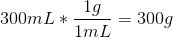2. Since the heat from the iron is being transferred to the water, we can say that the heat transfer is equal between both compounds. Since the heat is conserved in the system, we can set the two equations equal to one another.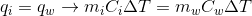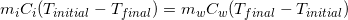Notice how the change in temperature for iron has been flipped in order to avoid a negative number.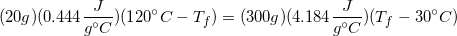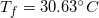Because water has a much higher heat capacity compared to iron, the temperature of the water is not changed significantly.

### Example Question #11 : Thermodynamics

Which of these conditions will result in a spontaneous reaction?

Exothermic reactions with reactants going from gaseous to liquid state

Exothermic reactions with reactants going from liquid to gaseous state

Endothermic reactions with reactants going from gaseous to liquid state

Endothermic reactions with reactants going from liquid to gaseous state

All exothermic reactions

Exothermic reactions with reactants going from liquid to gaseous state

Explanation:

To determine whether a reaction will occur spontaneously, one needs to look at whether the Gibbs free energy will decrease. In order for Gibbs free energy to decrease, the combination of the heat of the reaction and the change in entropy must give a negative Gibbs free energy. The equation is: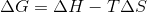If a reaction is exothermic, the heat of the reaction is negative. If the reactants go from liquid to gaseous state, the entropy increases (is positive). When the enthalpy is negative and the entropy is positive, the equation must always give a negative solution. This leads to a negative Gibbs free energy, so the reaction will be spontaneous.

### Example Question #12 : Thermodynamics

Which of the following reaction conditions cannot result in a spontaneous reaction?

Exothermic reactions in which reactants go from liquid to gaseous state

Endothermic reactions in which reactants go from gaseous to liquid state

Endothermic reactions in which reactants go from liquid to gaseous state

Exothermic reactions in which reactants go from gaseous to liquid state

Exothermic reactions in which reactants go from liquid to solid state

Endothermic reactions in which reactants go from gaseous to liquid state

Explanation:

To determine whether a reaction will occur spontaneously, one needs to look at whether the Gibbs free energy will decrease. In order for Gibbs free energy to decrease, the combination of the heat of the reaction and the change in entropy must give a negative Gibbs free energy. The equation is:If a reaction is endothermic, the heat of the reaction is positive. If the reactants go from gaseous to liquid state, the entropy decreases (is negative). When the enthalpy is positive and the entropy is negative, the equation must always give a positive solution. This leads to a positive Gibbs free energy, so the reaction can never be spontaneous.

### Example Question #13 : Thermodynamics

Which of the following values must be negative in order for a reaction to be spontaneous?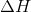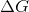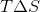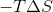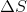Explanation:

In order to determine if a reaction is spontaneous, one needs to look at, which is the change in Gibbs free energy. The formula to find change in Gibbs free energy is:andcan have positive or negative values (under certain conditions), butmust always be negative in order for the reaction to be spontaneous.

### Example Question #1 : Endothermic And Exothermic Reactions

What type of energy conversion occurs during an endothermic reaction?

Kinetic energy to chemical energy

Chemical energy to kinetic energy

Nuclear energy to chemical energy

Kinetic energy to potential energy

Temperature to chemical energy

Kinetic energy to chemical energy

Explanation:

In an endothermic reaction, heat is absorbed and converted into chemical energy. Heat is the sum of molecular kinetic energy in a sample, so kinetic energy is converted into chemical energy. Note that temperature is a measure of heat, and is not a type of energy in itself.

### Example Question #2 : Endothermic And Exothermic Reactions

What will happen to the rate of an exothermic reaction if the temperature is increased?

It will be unaffected

It will increase

It will decrease

It depends on the initial concentrations of the reactants

It will increase

Explanation:

The rate of a reaction will always increase with temperature.

Do not confuse the rate at which a reaction proceeds with the final equilibrium of the reaction. Yes, increasing the temperature of an exothermic reaction will push the equilibrium towards the reactant's side, but this equilibrium will be achieved much more quickly at higher temperatures. Kinetics and thermodynamics are two completely different ways to observe a reaction.

### Example Question #3 : Endothermic And Exothermic Reactions

If a reaction is endothermic and occurs spontaneously, which of the following is not true?

ΔH > 0

ΔS > 0

K = 0

ΔG < 0

K = 0

Explanation:

ΔH is always positive for an endothermic reaction, and ΔG is always negative for a spontaneous reaction. Given the equation delta G = ΔH – T(ΔS), T(ΔS) is positive, so ΔS is positive. We do not know anything about the equilibrium of the reaction.

### Example Question #4 : Endothermic And Exothermic Reactions

If a reaction has a negative entropy and a negative enthalpy value, which of the following terms describes the energy of this reaction?